## Information Diffusion in Networks

 Understanding the process by which a contagion disseminates throughout a network is of a great importance in many real world applications. The sophistication of the inference approach depends on the type of information we want to extract as well as the amount of observation that is available to us. In this paper, we analyze scenarios in which not only the underlying net- work structure (parental relationships and link strengths) needs to be detected, but also the infection times must be estimated. We assume that our only observation of the diffusion process is a set of time series, one for each node of the network, which exhibit changepoints when an infection occurs. After formulating a model to describe the contagion, and selecting appropriate prior distributions, we seek to find the set of model parameters that best explains our observations. Modeling the problem in a Bayesian framework, we exploit Monte Carlo Markov Chain, Sequential Monte Carlo, and time series analysis techniques to develop batch and online inference algorithms. We evaluate the performance of our proposed algorithms via numerical simulations of synthetic network contagions and analysis of real-world datasets.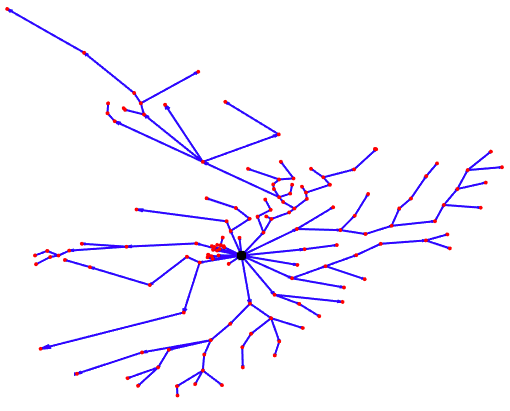Network Inference with Perfect Cascade Observations:

Most of the earlier work exploring diffusion network inference techniques assume that cascades were perfectly observed i.e. the infection times are exactly known. Given the infection times, they infer the parental relationships and estimates the link strengths that maximize likelihood of the observed data.

Network Inference without Perfect Cascade Observations:

Unlike the first category of related works, studies of this group investigate scenarios in which the cascade trace is not directly observable or is at least partially missing. Two important examples of such scenarios are the outbreak of a contagious disease (with nodes being geographical regions) and the impact of external events on the stock returns of different assets. These studies either assume that some portion of the cascade data is observable and try to infer the causality structure from this portion or infer the structure using some other observable property of the cascade without inferring the cascade trace itself.

Changepoint Detection Methods:

Another sizeable, related body of literature addresses detecting abrupt changes in the statistical structure of multiple time series. The moments in time that divide time series into distinct homogeneous segments are referred to as changepoints. Most changepoint detection, or time series segmentation, methods strive to detect single and multiple changepoints in univariate or independent multivariate time series. In these models, there is no notion of a diffusion process; they capture contemporaneous correlation structure.

We consider a set of N nodes that are exposed to a contagion C. We assume that C originates in a subset of nodes and is transmissible to other nodes of the network.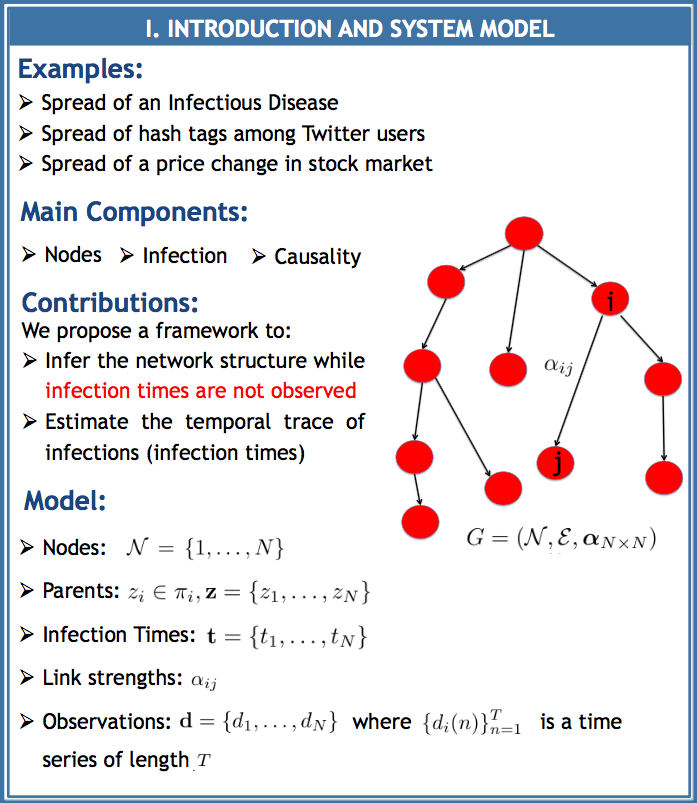Assuming that the entire length of data signals is available, we develop a batch (offline) inference algorithm based on Gibbs Sampling.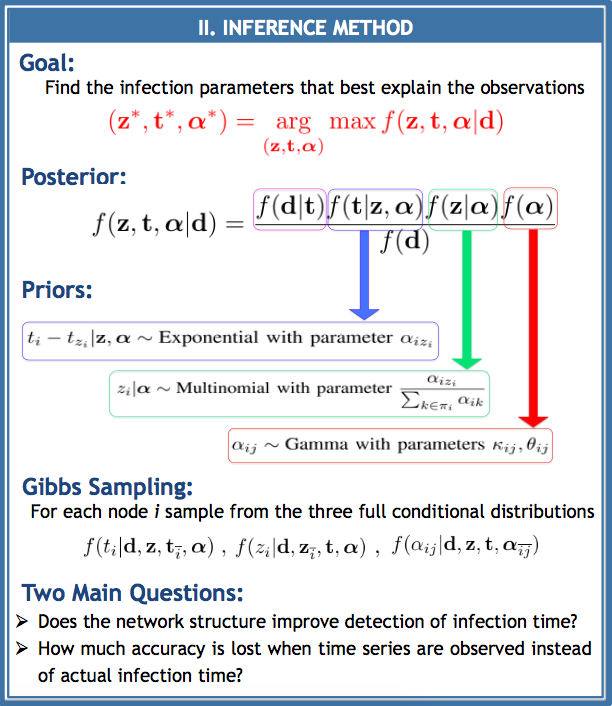Synthetic Data:

We generate a dataset based on the described model. In order to evaluate the performance of our proposed inference approach, two main questions should be answered: (1) Does the network structure improve detection of infection times? (2) How much accu- racy is lost in terms of detecting the parents and estimating link strengths when time series are observed instead of the actual infection times?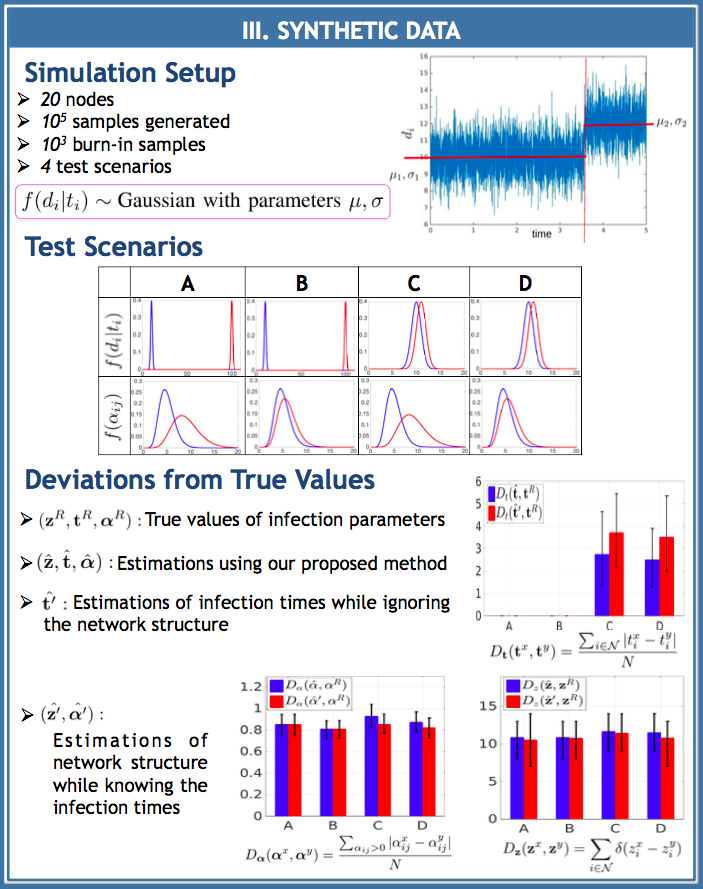Real Data:

We study the outbreak of Avian Influenza (H5N1 HPAI). The following map shows the observed locations of reported infections for both domestic and wild bird species for the period of January 2004 to February 2016. We divide the observation points to eight main regions using K-means clustering and generate a time series for each region. The value of this time series at day n denotes the number of separate locations the region  in which the disease was reported on that day.  We model the number of observations in each region by a Poisson distribution. Thelink strength parameters and of equation are derived by fitting a gamma distribution to the inverse of distances between observation points of regions. The following figure shows the time series for the eight regions. Regions R5 and R8 are the first regions in which the disease is observed. The first infections for these regions were reported on the same day, so we assume that they were both sources of the infection. We infer the infection parameters for the period 2004-2007 by generating 10samples and discarding the first 10ones. The green line in Figure 4 shows the end of the study period. Region R4 has almost no reported infections for this period so we exclude it when estimating the underlying infec- tion graph. The detected infection times are shown by red vertical lines. Here are the four most probable configurations of the infection network and their percentages among generated samples. The edge weights in these graphs are estimated link strengths.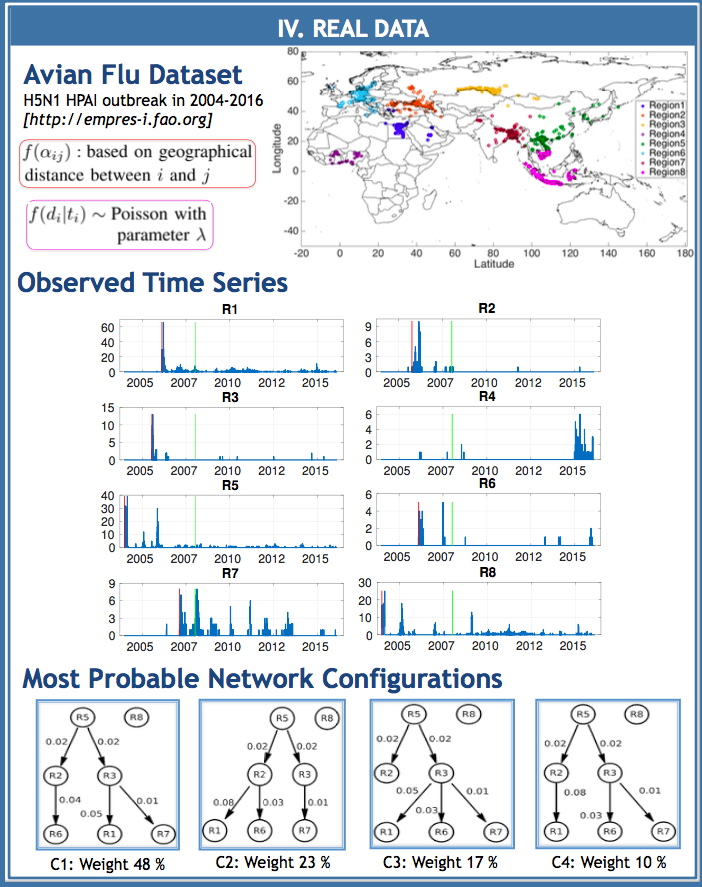Ph.D. Student: Shohreh Shaghaghian

Faculty: Prof. Mark Coates

"Bayesian Inference of Diffusion Networks with Unknown Infection Times", IEEE Statistical Signal Procession (SSP), Spain, Jun, 2016.